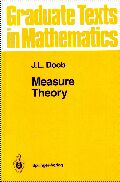If I were a Springer-Verlag Graduate Text in Mathematics, I would be J.L. Doob's Measure Theory.I am different from other books on measure theory in that I accept probability theory as an essential part of measure theory. This means that many examples are taken from probability; that probabilistic concepts such as independence, Markov processes, and conditional expectations are integrated into me rather than being relegated to an appendix; that more attention is paid to the role of algebras than is customary; and that the metric defining the distance between sets as the measure of their symmetric difference is exploited more than is customary. Which Springer GTM would you be? The Springer GTM Test